Lec 20 - Linear Independence & Basis

Friday, February 17, 2012

9:29 AM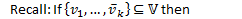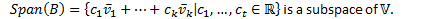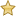Linear Independence

Definition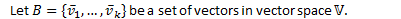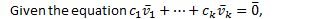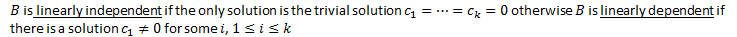Eg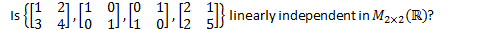Solve the equation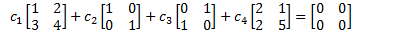We get the following homogeneous system of linear equations: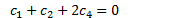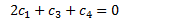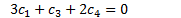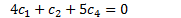Row reduce the coefficient matrix: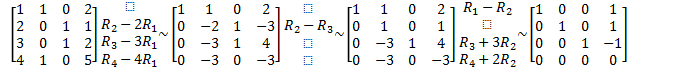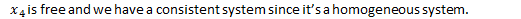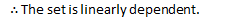Basis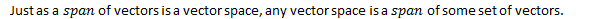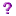What is the smallest spanning set of a vector space?Theorem: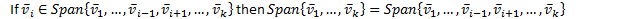Proof: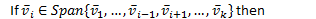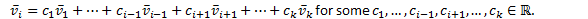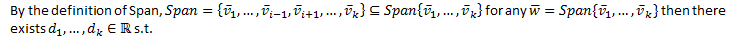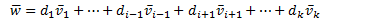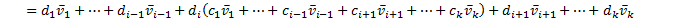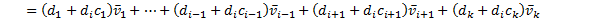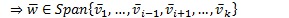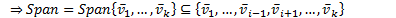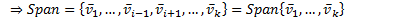Theorem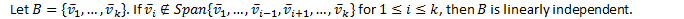Proof:

Prove the contrapositive: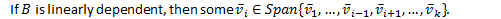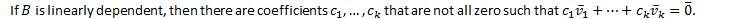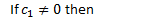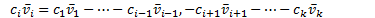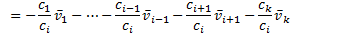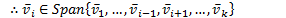Both theorems together imply the smallest spanning set of any vector space is linearly independent.

Definition: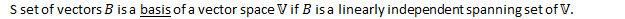Eg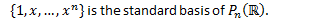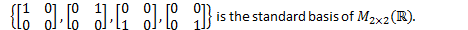Eg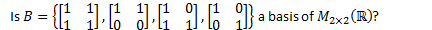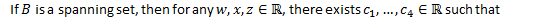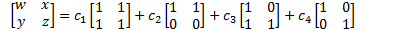This gives a system of linear equations: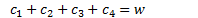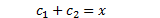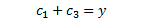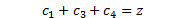Row reduce the coefficient matrix: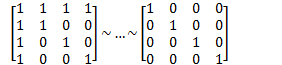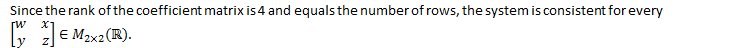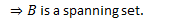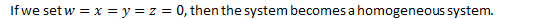Since the rank of coefficient matrix must equal to the number of columns, the homogeneous system has a unique solution (the trivial one).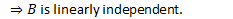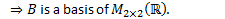Created by Tim Pei with Microsoft OneNote 2010
One place for all your notes and information